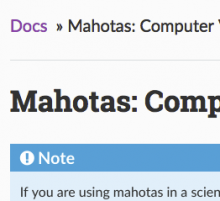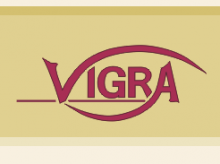# slic superpixels

## Mahotas

Description

This library gives the numpy-based infrastructure functions for image processing with a focus on bioimage informatics. It provides image filtering and morphological processing as well as feature computation (both image-level features such as Haralick texture features and SURF local features). These can be used with other Python-based libraries for machine learning to build a complete analysis pipeline.

Mahotas is appropriate for users comfortable with programming or builders of end-user tools.

==== Strengths

The major strengths are in speed and quality of documentation. Almost all of the functionality is implemented in for multiple dimensions. It can be used with other Python packages which provide additional functionality.

Mahotas and all packages on which it relies are open-source.

has function## VIGRA

Description

VIGRA is a free C++ and Python library that provides fundamental image processing and analysis algorithms. Its generic architecture allows it to be used in many different application contexts and ecosystems. It is designed as an intelligent library (using the C++ template mechanism) which allows users to write code at a fairly high level of abstraction and optimizes away the abstraction overhead upon compilation. It can therefore work efficiently on very large data and forms the basis of ilastik and CellCognition.

Strengths: open source, high quality algorithms, unlimited array dimension, arbitrary pixel types and number of channels, high speed, well tested, very flexible, easy-to-use Python bindings, support for many common file formats (including HDF5)

Limitations: no GUI, C++ not suitable for everyone, BioFormats not supported, parallelization requires external control

Images and Multi-dimensional Arrays: templated image data structures for arbitrary pixel types, fixed-size vectors multi-dimensional arrays for arbitrary high dimensions pre-instantiated images with many different scalar and vector valued pixel types (byte, short, int, float, double, complex, RGB, RGBA etc.) 2-dimensional image iterators, multi-dimensional iterators for arbitrary high dimensions, adapters for various image and array subsets

input/output of many image file formats: Windows BMP, GIF, JPEG, PNG, PNM, Sun Raster, TIFF (including 32bit integer, float, and double pixel types and multi-page TIFF), Khoros VIFF, HDR (high dynamic range), Andor SIF, OpenEXR input/output of images with transparency (alpha channel) into suitable file formats. comprehensive support for HDF5 (input/output of arrays in arbitrary dimensions)

continuous reconstruction of discrete images using splines: Just create a SplineImageView of the desired order and access interpolated values and derivative at any real-valued coordinate.

Image Processing: STL-style image processing algorithms with functors (e.g. arithmetic and algebraic operations, gamma correction, contrast adaptation, thresholding), arbitrary regions of interest using mask images image resizing using resampling, linear interpolation, spline interpolation etc.

geometric transformations: rotation, mirroring, arbitrary affine transformations automated functor creation using expression templates

color space conversions: RGB, sRGB, R'G'B', XYZ, Lab*, Luv*, Y'PbPr, Y'CbCr, Y'IQ, and Y'UV real and complex Fourier transforms in arbitrary dimensions, cosine and sine transform (via fftw) noise normalization according to Förstner computation of the camera magnitude transfer function (MTF) via the slanted edge technique (ISO standard 12233)

Filters: 2-dimensional and separable convolution, Gaussian filters and their derivatives, Laplacian of Gaussian, sharpening etc. separable convolution and FFT-based convolution for arbitrary dimensional data resampling convolution (input and output image have different size) recursive filters (1st and 2nd order), exponential filters non-linear diffusion (adaptive filters), hourglass filter total-variation filtering and denoising (standard, higer-order, and adaptive methods)

tensor image processing: structure tensor, boundary tensor, gradient energy tensor, linear and non-linear tensor smoothing, eigenvalue calculation etc. (2D and 3D) distance transform (Manhattan, Euclidean, Checker Board norms, 2D and 3D) morphological filters and median (2D and 3D) Loy/Zelinsky symmetry transform Gabor filters

Segmentation: edge detectors: Canny, zero crossings, Shen-Castan, boundary tensor corner detectors: corner response function, Beaudet, Rohr and Förstner corner detectors tensor based corner and junction operators

region growing: seeded region growing, watershed algorithm

Image Analysis: connected components labeling (2D and 3D) detection of local minima/maxima (including plateaus, 2D and 3D) tensor-basesd image analysis (2D and 3D) powerful incremental computation of region and object statistics

3-dimensional Image Processing and Analysis: point-wise transformations, projections and expansions in arbitrary high dimensions all functors (e.g. regions statistics) readily apply to higher dimensional data as well separable convolution and FFT-based convolution filters, resizing, morphology, and Euclidean distance transform for arbitrary dimensional arrays (not just 3D) connected components labeling, seeded region growing, watershed algorithm for volume data

Machine Learning: random forest classifier with various tree building strategies variable importance, feature selection (based on random forest) unsupervised decomposition: PCA (principle component analysis) and pLSA (probabilistic latent semantic analysis)

Mathematical Tools: special functions (error function, splines of arbitrary order, integer square root, chi square distribution, elliptic integrals) random number generation rational and fixed point numbers quaternions polynomials and polynomial root finding matrix classes, linear algebra, solution of linear systems, eigen system computation, singular value decomposition

optimization: linear least squares, ridge regression, L1-constrained least squares (LASSO, non-negative LASSO, least angle regression), quadratic programming

Inter-language support: Python bindings in both directions (use Python arrays in C++, call VIGRA functions from Python) Matlab bindings of some functions

has topic
has function